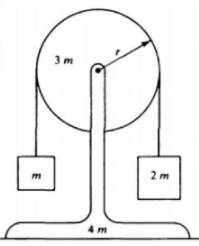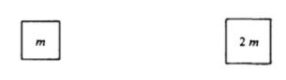# Problem: A pulley of mass 3m and radius r is mounted on frictionless bearings and supported by a stand of mass 4m at rest on a table as shown at right. The moment of inertia of this pulley about its axis is 1.5m2. Passing over the pulley is a massless cord supporting a block of mass m on the left and a block of mass 2m on the right. The cord does not slip on the pulley, so after the block-pulley system is released from rest, the pulley begins to rotate.a. On the diagrams below, draw and label all the forces acting on each block.b. Use the symbols identified in part (a) to write each of the following: i. The equations of translational motion (Newton's 2nd law) for each of the two blocks, and ii. The analogous equation for the rotational motion of the pulley. C. Solve the equations in part (b) for the acceleration of the two blocks. d. Determine the tension in the segment of the cord attached to the block of mass m. e Determine the total kinetic energy gain of the system after 1 second has passed. f. Determine the normal force exerted on the apparatus by the table while the blocks are in motion.

###### FREE Expert Solution

We'll use Newton's second law:

$\overline{){\mathbf{\Sigma }}{\mathbf{F}}{\mathbf{=}}{\mathbf{m}}{\mathbf{a}}}$

a.

The forces that will be acting on the mass, m are:

- Tension, T1 on the upward direction.

- The weight of the mass, mg downwards.

80% (338 ratings)###### Problem Details

A pulley of mass 3m and radius r is mounted on frictionless bearings and supported by a stand of mass 4m at rest on a table as shown at right. The moment of inertia of this pulley about its axis is 1.5m2. Passing over the pulley is a massless cord supporting a block of mass m on the left and a block of mass 2m on the right. The cord does not slip on the pulley, so after the block-pulley system is released from rest, the pulley begins to rotate.a. On the diagrams below, draw and label all the forces acting on each block.b. Use the symbols identified in part (a) to write each of the following:

i. The equations of translational motion (Newton's 2nd law) for each of the two blocks, and

ii. The analogous equation for the rotational motion of the pulley.

C. Solve the equations in part (b) for the acceleration of the two blocks.

d. Determine the tension in the segment of the cord attached to the block of mass m.

e Determine the total kinetic energy gain of the system after 1 second has passed.

f. Determine the normal force exerted on the apparatus by the table while the blocks are in motion.